# Confidence Interval

It is an estimate of a range that might include a population parameterReviewed by

Manu Lakshmanan

Expertise: Management Consulting | Strategy & Operations

Updated:

September 30, 2023

## What is Confidence Interval?

In statistics, Confidence Interval (CI) is an estimate of a range that might include a population parameter. A sample parameter derived from the sampled data is used to find the unknown population parameter.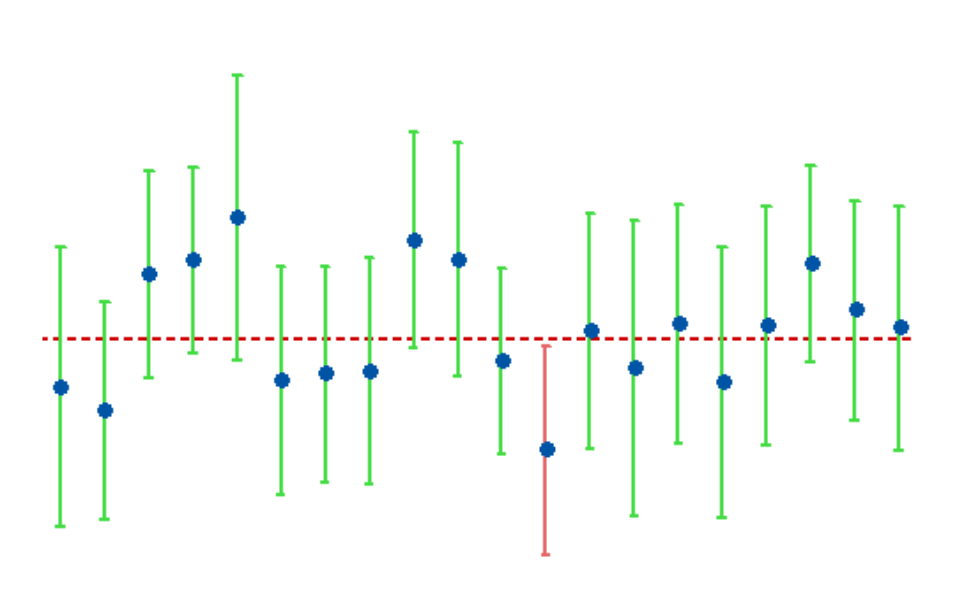In simple words, the probability of an estimate of how well a study's metric describes the behavior of your entire user population is provided by the confidence-interval calculation.

These intervals generally lie between the upper and lower bounds and are symbolized by percentage. These percentages demonstrate the confidence levels. The confidence level is the long-run fraction of related CIs that contain the parameter's real value.

The confidence level, sample size, and sample variability are all variables that have an impact on the CI's breadth. A bigger sample would result in a smaller confidence range if everything else remained constant.

Similar to how a broader confidence interval is produced by increased sample variability, a wider confidence interval is required by higher levels of confidence.

## Understanding Confidence Interval

Using the Confidence Interval, a statistician may assess how well research predicts the entire population's behavior. There must be a matching CI for every research outcome variable, including success, task duration, number of mistakes, and other parameters.

Although more desired and containing more information, narrow intervals typically call for bigger sample sizes. Because of this, it is doubtful that small research results would accurately reflect all users' behavior.

Where is it used? Researchers use this to assess the level of uncertainty in a sample variable value.

For instance, to determine how each sample could accurately reflect the real value of the population variable, a researcher randomly picks many samples from the same population and computes a confidence interval for each sample.

All of the generated datasets are unique, with some intervals including the real population parameter and others not.

## Confidence Levels

The proportion of potential samples that are anticipated to include the true population parameter, i.e., how frequently you repeat the experiment or resample the population in the same manner, to obtain an estimate that is close to it.

For example, if the value of the confidence level is 99%, then out of hundred samples are taken, 99 of them would have the parameter's true value. The commonly used levels are 90%, 95%, and 99%.

Because it affects your sample size and CI, the confidence level you choose is crucial. Your sample size needs to be greater the more confident you wish to be. The larger the Confidence Interval, the more confident you want to be.

## Different Confidence Intervals

There are two kinds of Confidence Intervals that can be used for estimating the mean:

• Z- Interval: When the sample size is greater than or equal to 30 and the standard deviation of the population is known, or the original population is normally distributed with the population standard deviation being known; at that point, the z-score is used.

• T- Interval: When Population standard deviation is unknown, and the original population is normally distributed, or the sample size is greater than or equal to 30, and the population standard deviation is unknown, the t-interval is used.

The Margin of Error (MOE) is an indicator of the extent of random sampling error in survey findings is the margin of error. A poll's ability to accurately represent the findings of a survey of the complete population should be questioned the greater the margin of error.

If the outcome measure has a positive variance, this means that the measure fluctuates, or the population was not entirely sampled.

The formula of Margin of Error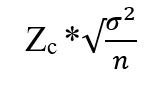Where,

Zc= is the critical value using the z-score and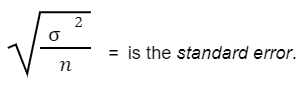But what is the purpose of CI? It is termed to be the measure of uncertainty. This method is often used for research-based projects like clinical trials. This can be represented as how "acceptable" the estimation of a trial is.

There are mainly two ways to derive the interval:

• Traditional approach: This is the historical/mathematical approach of solving the trial data by the use of a statistical T-test.

• Computerized approach: Computerized approach: This technique is by bootstrap resampling. Bradley Efron first defined this lucid yet powerful method. A single set of observations is used to generate several resamples (with replacement), and each resamples effect size is calculated.

## How to Interpret a Confidence Interval

Interpreting the intervals can sometimes be difficult as people phrase it in various ways. This can be assumed as that there is 99% confidence that the actual value of the parameter will fall within the upper limit and the lower limit in the future.

This does not mean:

• That 99% of the trial data lie within the Confidence Interval or

• A determined confidence level of 99% does not always indicate that there is a 99% chance that a trial parameter from a subsequent run of the experiment will fall within this range.

## How to Calculate Confidence Interval

The steps we can follow are:

1. Find the mean of the dataset.

2. Determine whether the standard deviation is known or unknown.

3. Obtain the standard deviation using the z-score or the t-test.

4. Calculate the upper and the lower bounds in accordance with the standard deviation

Examples: These illustrations are to be solved using the above-mentioned steps. These will include both using a z-score and t-test with different confidence levels.

1. Formula using z-score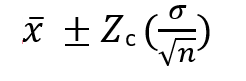Zc here is the critical value of the confidence level from the normal distribution.
The most commonly used critical values are as follows:

CONFIDENCE LEVEL CRITICAL VALUES
90% 1.645
95% 1.96
99% 2.575

Question: There are 100 cities listed for a survey of using radios by college students. The mean of this survey resulted in 3 cities, while the standard deviation was 0.5. Use this information to calculate a 95% confidence interval for the mean of the cities still using radios.

Solution: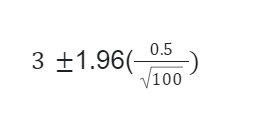Upper bound: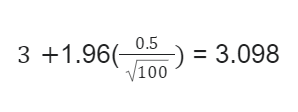Lower bound: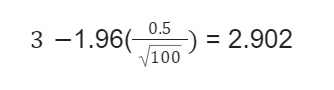The value of the upper end here would be 3.098, while the value of the lower end is 2.902

In this case, it can be assumed that the survey is 95% certain that the mean of the cities still using radios will fall between 2.902 and 3.098.

2. Formula using T-Interval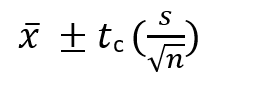T here is the critical value from the t-distribution, while is the standard deviation.

In this case, n is the sample size used to find out the degree of freedom(df).

The number of values in a statistic's final computation that is subject to change is referred to as its degrees of freedom.

df here is,

df = n-1 (n is the sample size)

Question: Suppose that a sample of 41 employees at a large company was asked how many hours a week were inefficient. The mean number of hours is 12.4, with a standard deviation of 5.1. Calculate a 90% confidence interval to estimate the mean of the time, which is inefficient per week.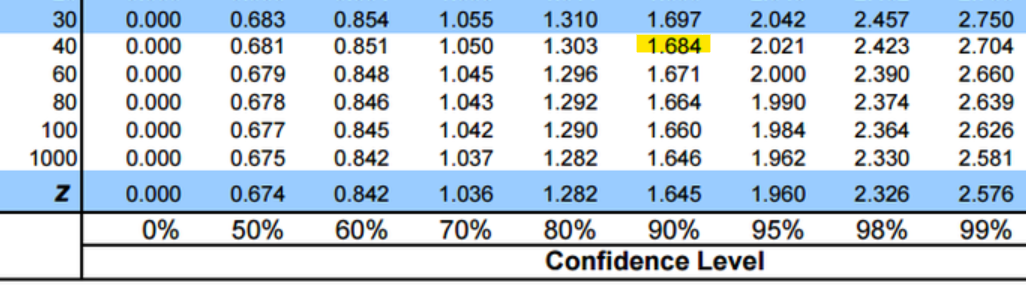Source: T Table - T Score Table - T Critical Value

Solution:

n = df = n-1

While calculating through t-interval, the critical value is found using the t-tableº.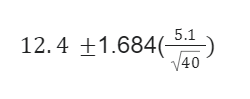Upper bound: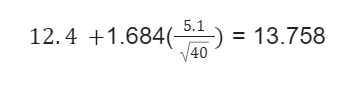Lower bound: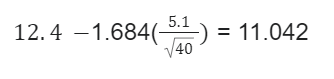In this case, it will be interpreted that the survey is 90% optimistic that the mean of the inefficient time will fall between 11.042 and 13.758.

Question: Suppose that there is a drug trial of 26 people for the side effects of the drug. The mean number of hours is 9.5, with a standard deviation of 5.1. Calculate a 99% confidence interval to estimate the mean of the time, which is inefficient per week.

Solution: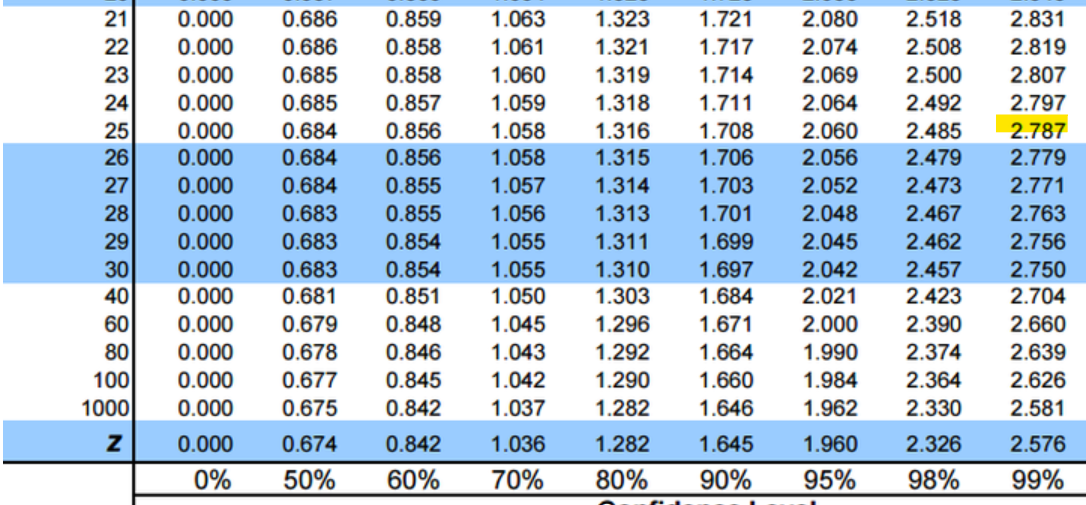Source: T Table - T Score Table - T Critical Value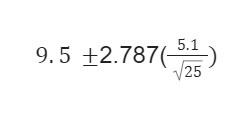Upper bound: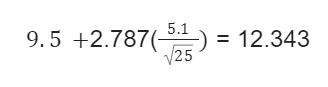Lower bound: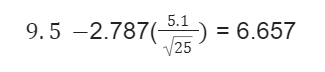Here, it will be interpreted that the survey is 99% certain that the mean of drug trialed people for examining the side-effects will fall between 6.657 and 12.343.

## What is Hypothesis testing?

The plausibility of a hypothesis is evaluated using sample statistics in a process known as hypothesis testing. A data-generating process or a wider population may be the source of such statistics.

Statistical analysts can assess a theory by measuring and examining a representative sample of the population being studied. Each analyst tests the null and alternative hypotheses using a random population sample.

For instance, a null hypothesis can state that the population means the return is equal to zero. For population parameters, the null hypothesis is frequently an equality hypothesis.

The alternative hypothesis is practically the opposite of a null hypothesis (e.g., the population means the return is not equal to zero). Since they contradict one other, neither can be true. However, one of the two hypotheses will always be true.

1. Significance Level

The significance level, commonly referred to as alpha or, is a gauge of how much evidence must be present in your sample for you to reject the null hypothesis and determine that the effect is statistically significant.

Before starting the experiment, the researcher chooses the degree of significance.

The chance of rejecting the null hypothesis when it is true is the significance level. A significance level of 0.05, for instance, denotes a 5% likelihood of drawing the incorrect conclusion that a difference exists when none really does.

Lower significance levels suggest that more convincing evidence is needed before you can rule out the null hypothesis.

2. One-Tail Testing

The critical area of a distribution in a one-tailed test is one-sided, meaning that it can either be more than or less than a certain number, but not both. The alternative hypothesis will be accepted instead of the null hypothesis if the sample under test falls into the one-sided critical region.

3. Two-Tail Testing

In statistics, a two-tailed test is a procedure that determines if a sample is larger than or less than a particular range of values by using a two-sided critical area of distribution. It is utilized in tests for statistical significance and null hypotheses.

The alternative hypothesis is adopted in place of the null hypothesis if any of the critical regions apply to the sample under test. This is calculated using the z-score to find the range of the significant regions.

## Confidence Interval Common Mistakes

Generally, it is believed that a 95 percent CI does not imply that 99 percent of the data in a random sample falls within these limitations. The range will almost certainly include the population mean, which is what it truly indicates.

Once the interval is constructed, the true parameter is either inside or outside the interval, and no one knows for certain where it falls. We can only state how frequently such intervals may include the genuine mean; this is what confidence level is all about.

This also does not imply that 95% of the sample data lie within the confidence interval. Here, there is a 95% certainty that the sample data might fall in the range of the interval.

The explanation for this error is rather minor. The important concept relating to a CI is that the probability utilized enters the picture with the technique used in calculating it that relates to the method used.

### Confidence Interval FAQs### Everything You Need To Master Valuation Modeling

To Help You Thrive in the Most Prestigious Jobs on Wall Street.